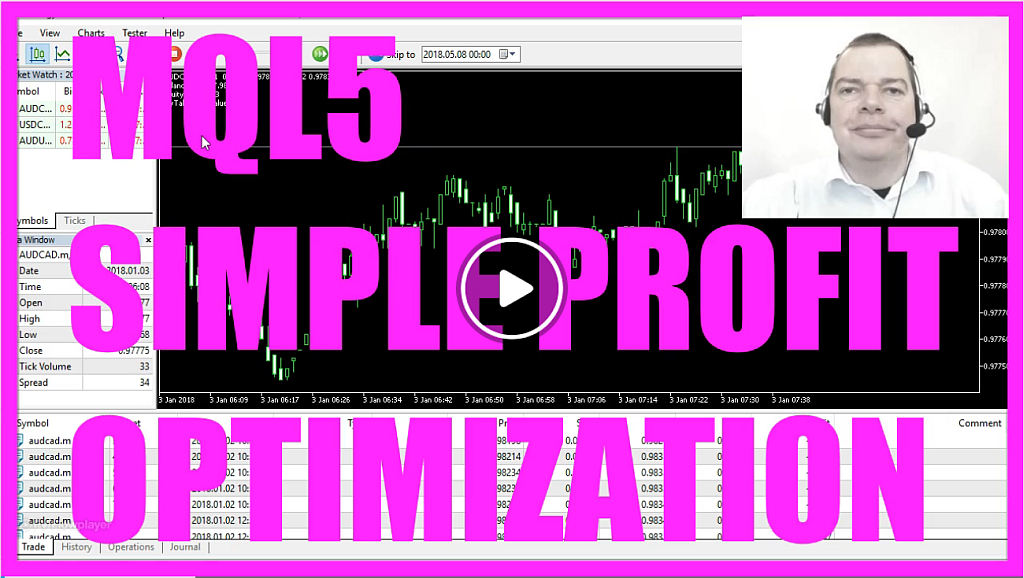In this video we want to test the take profit value, in our case we are just testing the 100 point take profit value here. Our little test Expert Advisor is just opening buy trades. Whenever the equity is equal or above the balance its opening lots of positions so please don’t do that on a real account.
Now we want to find out how we can create an Expert Advisor to test the take profit value in MQL5.
Now you should see the Metaeditor window here, and here you want to click on: “File/ New/ Expert Advisor (template)” from template, “Continue”, I will call this one: “SimpleTakeProfitTest”, click on “Continue”, “Continue” and “Finish”.
Now you can delete everything that is above the “OnTick” function and let’s remove the two comment lines here.
Okay.
First we need to create an instance of “CTrade”.
“CTrade” is included in the “Trade” library that comes with MQL5 and we will create a new instance called: “trade”. We also want create a user input variable that is done by using the modifier input, it’s an integer variable and we call it: “MyTakeProfitValue” and the initial value will be 100.
Now we need to calculate a few things; first we need to get the “Ask” price, that is done by using “SymbolInfoDouble” for the current symbol on the chart, we want to get “Symbol_ask” and by using “NormalizeDouble” and “_Digits” we make sure that we get 3 digits behind the dot for a 3 digit value and other currency pairs have 5 digits behind the dot and “_Digits” will give us the number of digits after the decimal point.
We also need the current account balance and we get that by using “AccountInfoDouble(ACCOUNT_BALANCE)”.
Now let’s calculate the equity – this is similar – we use “AccountInfoDoulbe(ACCOUNT_EQUITY)”.
Now we want to create a simple buy signal, when the equity is equal or above the balance; so if equity is greater or equal balance we are going to buy 10 micro lot of the currency pair.
Now here is our profit value variable that we have created as a user input and I will show you what we are going to do with it but before we do that we want to create a chart output by using the “Comment” function that will output the “Balance”, the “Equity” and the take profit value (MyTakeProfitValue) here.
Okay.
When you are done please click on the “Compile” button, we don’t have any errors here so now we can click here or press F4 to go back to Metatrader.
In Metatrader we click on: “View/ Strategy tester” or you can also press “CTRL and R”, let’s select the file: “SimpleTakeProfitTest.ex5”. I have randomly chosen a currency pair and the period here and now I want to disable the visualization mode here to speed things up and start a test. This should only take a few seconds, as soon as you see the “Results” tab the test finished. We have made 2.863 Dollars in this simple test. This is the graph, and now we click on: “Inputs” and we see that our take profit value (MyTakeProfitValue) can be changed here. Right now its 100, we could also change it to 200, start another test; this time we made a profit of 37.732 Dollars and 70 Cents.
Looks good for me but now comes the interesting part; I want to Optimize. So I choose “Optimization/ Fast Generic Based Algorithm” here, click on the “Inputs” tab, set the original value to 100, the start value to 100, the step value to 20 and the stop value to let say 300.
Now what this is going to do is: it’s starting with 100 take profit points and the next one will be increased by 20 points so the second one will have 120 take profit points and it will stop when we have reached 300 take profit points. We also need to mark the value we want to optimize for and start a new test without visualization.
Now you should see that several agents are going to calculate everything the first ones are already finished here and in a few seconds we should have all the results. Please remember this is just a quick test and now it’s finished.
Let’s sort by the take profit value (MyTakeProfitValue) here and you will see that we made the best profit with the take profit value of 240 points.
We could also change the step value here, let’s say we want to test with the step value of 10 so now the first one will be 100 points, the next one 110 points, the third one 120 points and so on.
This is a quick way how to test the optimal value for your take profit, we can also sort by the profit column here and here we see we made a profit of 109.502 Dollars with a take profit value of 250 points.
Now you know how to create an Expert Advisor to test the perfect take profit value and you have coded it yourself with a few lines of MQL5 code.